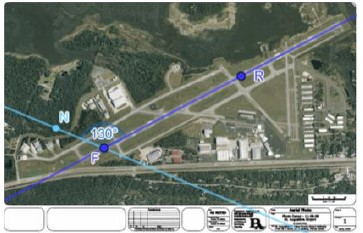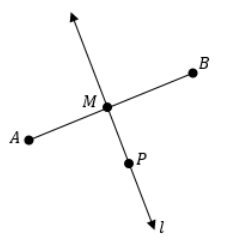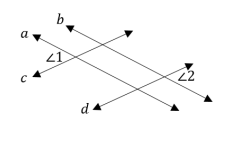# MA.912.GR.1.1Export Print
Prove relationships and theorems about lines and angles. Solve mathematical and real-world problems involving postulates, relationships and theorems of lines and angles.

### Clarifications

Clarification 1: Postulates, relationships and theorems include vertical angles are congruent; when a transversal crosses parallel lines, the consecutive angles are supplementary and alternate (interior and exterior) angles and corresponding angles are congruent; points on a perpendicular bisector of a line segment are exactly those equidistant from the segment’s endpoints.

Clarification 2: Instruction includes constructing two-column proofs, pictorial proofs, paragraph and narrative proofs, flow chart proofs or informal proofs.

Clarification 3: Instruction focuses on helping a student choose a method they can use reliably.

General Information
Subject Area: Mathematics (B.E.S.T.)
Strand: Geometric Reasoning
Status: State Board Approved

## Benchmark Instructional Guide

### Terms from the K-12 Glossary

• Angle
• Congruent
• Corresponding Angles
• Supplementary Angles
• Transversal
• Vertical

### Vertical Alignment

Previous Benchmarks

Next Benchmarks

### Purpose and Instructional Strategies

In grade 3, students described and identified line segments, rays, perpendicular lines and parallel lines. In grade 4, students classified and solved problems involving acute, right, obtuse, straight and obtuse angle measures. In grade 8, students solved problems involving supplementary, complementary, adjacent and vertical angles. In Geometry, students prove relationships and theorems and solve problems involving lines and angle measure. In later courses, students will study lines and angles in two and three dimensions using vectors and they will use radians to measure angles.
• While focus of this benchmark are those postulates, relationships and theorems listed in Clarification 1, instruction includes other definitions, postulates, relationships or theorems such as the midpoint of a segment, angle bisector, segment bisector, perpendicular bisector, the angle addition postulate and the segment addition postulate. Additionally, some postulates and theorems have a converse (i.e., if conclusion, then hypothesis) that can be included.
• Instruction includes the connection to the Logic and Discrete Theory benchmarks when developing proofs. Additionally, with the construction of proofs, instruction reinforces the Properties of Operations, Equality and Inequality. (MTR.5.1
• For example, when proving that vertical angles are congruent, students must be able to understand and use the Substitution Property of Equality and the Subtraction Property of Equality.
• Instruction utilizes different ways students can organize their reasoning by constructing various proofs when proving geometric statements. It is important to explain the terms statements and reasons, their roles in a geometric proof, and how they must correspond to each other. Regardless of the style, a geometric proof is a carefully written argument that begins with known facts, proceeds from there through a series of logical deductions, and ends with the statement you are trying to prove. (MTR.2.1
• For examples of different types of proofs, please see MA.912.LT.4.8
• Instruction includes the connection to compass and straight edge constructions and how the validity of the construction is justified by a proof. (MTR.5.1
• Students should develop an understanding for the difference between a postulate, which is assumed true without a proof, and a theorem, which is a true statement that can be proven. Additionally, students should understand why relationships and theorems can be proven and postulates cannot.
• Instruction includes the use of hatch marks, hash marks, arc marks or tick marks, a form of mathematical notation, to represent segments of equal length or angles of equal measure in diagrams and images.
• Students should understand the difference between congruent and equal. If two segments are congruent  (i.e., PQMN),  then they have equivalent lengths (i.e., PQ = MN) and the converse is true. If two angles are congruent (i.e., ∠ABC ≅ ∠PQR), then they have equivalent angle measure (i.e., $m$ABC = $m$PQR) and the converse is true.
• Instruction includes the use of hands-on manipulatives and geometric software for students to explore relationships, postulates and theorems.
• For example, folding paper (e.g., patty paper) can be used to explore what happens with angle pairs when two parallel lines are cut by a transversal. Students can discuss the possible pairs of corresponding angles, alternate interior angles, alternate exterior angles and consecutive (same-side interior and same-side exterior) angles. (MTR.2.1, MTR.4.1)
• Problem types include mathematical and real-world context where students evaluate the value of a variable that will make two lines parallel; utilize two sets of parallel lines or more than two parallel lines; or write and solve equations to determine an unknown segment length or angle measure.
• Instruction for some relationships or postulates may be necessary in order to prove theorems.
• For example, to prove that consecutive angles are supplementary when a given transversal crosses parallel lines, students will need to know the postulate that states corresponding angles are congruent.
• For example, to prove or use the perpendicular bisector theorem, students will need to have knowledge of the definition of a perpendicular bisector of a segment and midpoint.

### Common Misconceptions or Errors

• Students may misuse the terms corresponding, alternate interior and alternate exterior as synonyms of congruent. To help address this conception, students should develop the understanding that these angles are congruent if and only if two parallel lines are cut by a transversal. Similarly, same-side angles are supplementary if and only if the two lines being cut by a transversal are parallel.

• Runways are identified by their orientation relative to Magnetic North as viewed by an approaching aircraft. Runway directions are always rounded to the nearest ten degrees and the zero in the “ones” column is never depicted (i.e., 170 degrees would be viewed as “17” and 20 degrees would be seen as “2”). The same runway has two names which are dependent on the direction of approach.
• Use the aerial of Northeast Florida Regional Airport in St. Augustine, FL to answer the questions below.• Part A. Flying to the runway from point F, the runway is Runway 13. This means the heading is 130° off magnetic north. Draw a line through point R to that goes to magnetic north, what is true about that line and line NF
• Part B. On the line drawn in Part A, draw and label a point, A
• Part C. Measure angle FRA
• Part E. What is the name of the runway when approaching from point R

• Part A. Given AB, use a compass and straightedge to construct line $l$ such that line $l$ and AB form 90° and the point of intersection, M, is the midpoint of AB
• Part B. Suppose that point P lies on line $l$ as shown below. What conjecture can be made about point P? Which endpoint of AB is closest to point P? Use a compass to test your conjecture.• Part C. What if a point, Q, was added to line $l$ Which endpoint of AB is closest to point Q How does this compare with your conjecture in Part B?
• Part D. How can the construction from Part A and the conjectures from Part B and Part C be used to prove that AP = BP given that line $l$ is the perpendicular bisector of AB and point P lies on line $l$?

### Instructional Items

Instructional Item 1
• What value of $x$ will make M the midpoint of PQ if  PM = 3$x$ − 1 and  PQ = 5$x$ + 3?

Instructional Item 2
• Two lines intersect at point P. If the measures of a pair of vertical angles are (2$x$ − 7)° and ($x$ + 13)°, determine $x$ and the measures of the other two angles?

Instructional Item 3
• Based on the figure below, complete a proof to prove that ∠1 ≅ ∠2 given that $a$ || $b$ and $c$ || $d$.*The strategies, tasks and items included in the B1G-M are examples and should not be considered comprehensive.

## Related Courses

This benchmark is part of these courses.
1200400: Foundational Skills in Mathematics 9-12 (Specifically in versions: 2014 - 2015, 2015 - 2022, 2022 and beyond (current))
1206310: Geometry (Specifically in versions: 2014 - 2015, 2015 - 2022, 2022 and beyond (current))
1206320: Geometry Honors (Specifically in versions: 2014 - 2015, 2015 - 2022, 2022 and beyond (current))
1206315: Geometry for Credit Recovery (Specifically in versions: 2014 - 2015, 2015 - 2022, 2022 and beyond (current))
7912065: Access Geometry (Specifically in versions: 2015 - 2022, 2022 and beyond (current))
1209315: Mathematics for ACT and SAT (Specifically in versions: 2022 and beyond (current))

## Related Access Points

Alternate version of this benchmark for students with significant cognitive disabilities.
MA.912.GR.1.AP.1: Use the relationships and theorems about lines and angles to solve mathematical or real-world problems involving postulates, relationships and theorems of lines and angles.

## Related Resources

Vetted resources educators can use to teach the concepts and skills in this benchmark.

## Formative Assessments

Finding Angle Measures - 1:

Students are asked to find the measures of angles formed by three concurrent lines and to justify their answers.

Type: Formative Assessment

Finding Angle Measures - 3:

Students are asked to find the measures of angles formed by two parallel lines and two transversals.

Type: Formative Assessment

Finding Angle Measures - 2:

Students are asked to find the measures of angles formed by two parallel lines and a transversal.

Type: Formative Assessment

Camping Calculations:

Students are asked to find the measure of an angle formed by the support poles of a tent using the properties of geometric shapes.

Type: Formative Assessment

Same Side Interior Angles:

Students are asked to describe and justify the relationship between same side interior angles.

Type: Formative Assessment

Justifying Angle Relationships:

Students are asked to describe and justify the relationship between corresponding angles and alternate interior angles.

Type: Formative Assessment

Proving the Alternate Interior Angles Theorem:

In a diagram involving two parallel lines and a transversal, students are asked to use rigid motion to prove that alternate interior angles are congruent.

Type: Formative Assessment

Constructions for Parallel Lines:

Students are asked to construct a line parallel to a given line through a given point.

Type: Formative Assessment

Equidistant Points:

Students are asked to prove that a point on the perpendicular bisector of a line segment is equidistant from the endpoints of the segment.

Type: Formative Assessment

Proving the Vertical Angles Theorem:

Students are asked to identify a pair of vertical angles in a diagram and then prove that they are congruent.

Type: Formative Assessment

## Lesson Plans

Accurately Acquired Angles:

Students will start the lesson by playing a game to review angle pairs formed by two lines cut by a transversal. Once students are comfortable with the angle pairs the teacher will review the relationships that are created once the pair of lines become parallel. The teacher will give an example of a proof using the angle pairs formed by two parallel lines cut by a transversal. The students are then challenged to prove their own theorem in groups of four. The class will then participate in a Stay and Stray to view the other group's proofs. The lesson is wrapped up through white board questions answered within groups and then as a whole class.

Type: Lesson Plan

What's the Point? Part 1:

This is a patty paper-folding activity where students measure and discover the properties of the point of concurrency of the perpendicular bisectors of the sides of a triangle.

Type: Lesson Plan

## Original Student Tutorial

Angle UP: Player 1:

Explore the construction processes for constructing an angle bisector, copying an angle and constructing a line parallel to a given line through a point not on the line using a variety of tools in this interactive, retro video game-themed tutorial.

NOTE: This tutorial uses both the angle bisector construction and the construction to copy an angle as an extension opportunity to also construct a line parallel to a given line through a point not on the line. Students also learn to identify corresponding angles created when a transversal crosses parallel lines, and discover using Geogebra that these angles are congruent.

Type: Original Student Tutorial

## MFAS Formative Assessments

Camping Calculations:

Students are asked to find the measure of an angle formed by the support poles of a tent using the properties of geometric shapes.

Constructions for Parallel Lines:

Students are asked to construct a line parallel to a given line through a given point.

Equidistant Points:

Students are asked to prove that a point on the perpendicular bisector of a line segment is equidistant from the endpoints of the segment.

Finding Angle Measures - 1:

Students are asked to find the measures of angles formed by three concurrent lines and to justify their answers.

Finding Angle Measures - 2:

Students are asked to find the measures of angles formed by two parallel lines and a transversal.

Finding Angle Measures - 3:

Students are asked to find the measures of angles formed by two parallel lines and two transversals.

Justifying Angle Relationships:

Students are asked to describe and justify the relationship between corresponding angles and alternate interior angles.

Proving the Alternate Interior Angles Theorem:

In a diagram involving two parallel lines and a transversal, students are asked to use rigid motion to prove that alternate interior angles are congruent.

Proving the Vertical Angles Theorem:

Students are asked to identify a pair of vertical angles in a diagram and then prove that they are congruent.

Same Side Interior Angles:

Students are asked to describe and justify the relationship between same side interior angles.

## Original Student Tutorials Mathematics - Grades 9-12

Angle UP: Player 1:

Explore the construction processes for constructing an angle bisector, copying an angle and constructing a line parallel to a given line through a point not on the line using a variety of tools in this interactive, retro video game-themed tutorial.

NOTE: This tutorial uses both the angle bisector construction and the construction to copy an angle as an extension opportunity to also construct a line parallel to a given line through a point not on the line. Students also learn to identify corresponding angles created when a transversal crosses parallel lines, and discover using Geogebra that these angles are congruent.

## Student Resources

Vetted resources students can use to learn the concepts and skills in this benchmark.

## Original Student Tutorial

Angle UP: Player 1:

Explore the construction processes for constructing an angle bisector, copying an angle and constructing a line parallel to a given line through a point not on the line using a variety of tools in this interactive, retro video game-themed tutorial.

NOTE: This tutorial uses both the angle bisector construction and the construction to copy an angle as an extension opportunity to also construct a line parallel to a given line through a point not on the line. Students also learn to identify corresponding angles created when a transversal crosses parallel lines, and discover using Geogebra that these angles are congruent.

Type: Original Student Tutorial

## Parent Resources

Vetted resources caregivers can use to help students learn the concepts and skills in this benchmark.